# xline

Vertical line with constant x-value

## Syntax

xline(xvalue)
xline(xvalue,LineSpec)
xline(xvalue,LineSpec,label)
xline(___,Name,Value)
xline(ax,___)
xl = xline(___)

## Description

example

xline(xvalue) creates a constant vertical line at the specified x-value in the current axes. For example, xline(2) creates a line at x = 2.

example

xline(xvalue,LineSpec) specifies either the line style, the line color, or both. For example, '-.' creates a dash-dot line, 'b' creates a blue line, and '-.b' creates a blue dash-dot line.

example

xline(xvalue,LineSpec,label) adds the specified label to the line.

example

xline(___,Name,Value) specifies constant line properties using one or more name-value pair arguments. For a list of properties, see ConstantLine Properties. Specify name-value pairs after all other input arguments.

example

xline(ax,___) creates the line in the specified axes instead of the current axes. To determine the current axes use gca.

example

xl = xline(___) returns the ConstantLine object created. Use xl to modify or query properties of the line after it is created.

## Examples

collapse all

Create a vertical line at x = 3.

xline(3);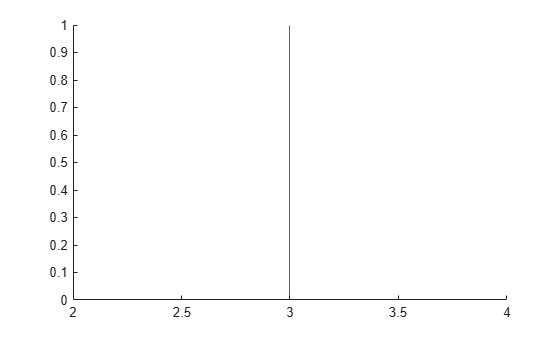Create a red dashed line at x = 5.

xline(5,'--r');To create a line with a label, you must also specify the line style. The default line style is a solid line, '-'.

x = linspace(0,6,100); y = exp(x); plot(x,y) xline(4.5,'-',{'Acceptable','Limit'});Create a vertical dash-dot line with both a label and a display name for a legend.

y = [3187 2693 1771 1826 1958 3222 1645]; barh(y,'DisplayName','Daily Sales') xl = xline(2328,'-.','Average','DisplayName','Average Sales');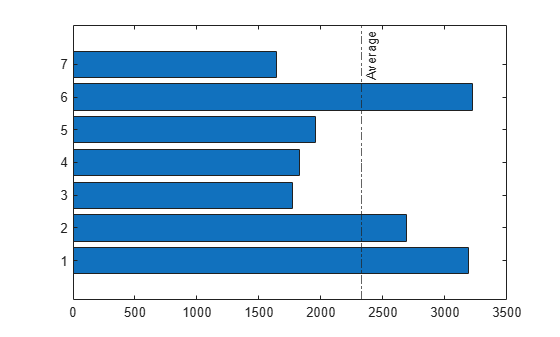Adjust the vertical and horizontal alignment of the line, then display the legend.

xl.LabelVerticalAlignment = 'middle'; xl.LabelHorizontalAlignment = 'center'; legend('show');Call the tiledlayout function to create a 2-by-1 tiled chart layout. Call the nexttile function to create the axes objects ax1 and ax2. Then plot into each of the axes. Add a dotted vertical line and label to each plot by passing the axes to the xline function.

tiledlayout(2,1) ax1 =nexttile; x = linspace(0,2*pi,200); y1 = cos(x); plot(ax1,x,y1) ax2 = nexttile; x = linspace(0,2*pi,200); y2 = sin(x); plot(ax2,x,y2) xlcosine = xline(ax1,pi/2,':','cos(\pi/2)=0'); xlsine = xline(ax2,pi,':','sin(\pi)=0');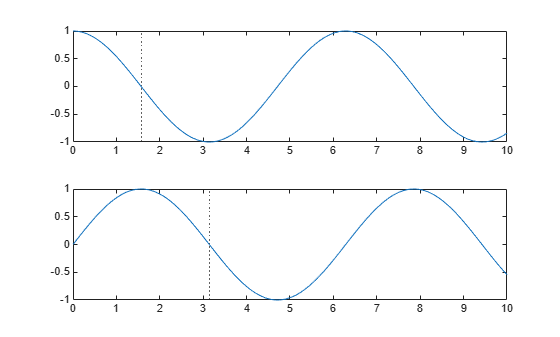## Input Arguments

collapse all

Location of the vertical line on the x-axis, specified as a scalar. You can specify xvalue as a numeric, categorical, datetime, or duration value. However, the type of value you specify must match the data type for the values along the x-axis.

Example: xline(12.4) plots the line at x = 12.4.

Example: xline(D(3)) plots the line at the value of the third element in the array.

Example: xline(categorical({'orange'})) plots the line at the category value 'orange'.

Line style, marker, and color, specified as a character vector or string containing symbols. The symbols can appear in any order. You do not need to specify all three characteristics (line style, marker, and color). For example, if you omit the line style and specify the marker, then the plot shows only the marker and no line.

Example: '--or' is a red dashed line with circle markers

Line StyleDescription
-Solid line
--Dashed line
:Dotted line
-.Dash-dot line
MarkerDescription
'o'Circle
'+'Plus sign
'*'Asterisk
'.'Point
'x'Cross
'_'Horizontal line
'|'Vertical line
's'Square
'd'Diamond
'^'Upward-pointing triangle
'v'Downward-pointing triangle
'>'Right-pointing triangle
'<'Left-pointing triangle
'p'Pentagram
'h'Hexagram
ColorDescription

y

yellow

m

magenta

c

cyan

r

red

g

green

b

blue

w

white

k

black

Target axes, specified as an Axes object. Use this argument if you want xline to plot in axes other than the current axes.

Line label, specified as a character vector, cell array of character vectors, string array, or numeric array. To create a multiline label, use a string array or a cell array of character vectors.

Example: xline(-pi/2,':','Lower Bound')

Example: xline(4,'-',{'first','second','third'})

### Name-Value Pair Arguments

Specify optional comma-separated pairs of Name,Value arguments. Name is the argument name and Value is the corresponding value. Name must appear inside quotes. You can specify several name and value pair arguments in any order as Name1,Value1,...,NameN,ValueN.

Example: xline(5,'-','Max','LabelVerticalAlignment','middle') specifies the vertical alignment of the label as 'middle'.

Note

The properties listed here are only a subset. For a complete list, see ConstantLine Properties.

Line color, specified as an RGB triplet, a hexadecimal color code, a color name, or a short name.

For a custom color, specify an RGB triplet or a hexadecimal color code.

• An RGB triplet is a three-element row vector whose elements specify the intensities of the red, green, and blue components of the color. The intensities must be in the range [0,1]; for example, [0.4 0.6 0.7].

• A hexadecimal color code is a character vector or a string scalar that starts with a hash symbol (#) followed by three or six hexadecimal digits, which can range from 0 to F. The values are not case sensitive. Thus, the color codes '#FF8800', '#ff8800', '#F80', and '#f80' are equivalent.

Alternatively, you can specify some common colors by name. This table lists the named color options, the equivalent RGB triplets, and hexadecimal color codes.

Color NameShort NameRGB TripletHexadecimal Color CodeAppearance
'red''r'[1 0 0]'#FF0000''green''g'[0 1 0]'#00FF00''blue''b'[0 0 1]'#0000FF''cyan' 'c'[0 1 1]'#00FFFF''magenta''m'[1 0 1]'#FF00FF''yellow''y'[1 1 0]'#FFFF00''black''k'[0 0 0]'#000000''white''w'[1 1 1]'#FFFFFF''none'Not applicableNot applicableNot applicableNo color

Here are the RGB triplets and hexadecimal color codes for the default colors MATLAB® uses in many types of plots.

[0 0.4470 0.7410]'#0072BD'[0.8500 0.3250 0.0980]'#D95319'[0.9290 0.6940 0.1250]'#EDB120'[0.4940 0.1840 0.5560]'#7E2F8E'[0.4660 0.6740 0.1880]'#77AC30'[0.3010 0.7450 0.9330]'#4DBEEE'[0.6350 0.0780 0.1840]'#A2142F'Example: 'g'

Example: [0.6 0.2 0.5]

Example: '#D2F9A7'

Line width, specified as a positive value in points.

Legend label, specified as a character vector or string scalar. The legend does not display until you call the legend command. If you do not specify the text, then legend sets the label using the form 'dataN'.

Horizontal alignment of the label with respect to the line, specified as one of the options in the table.

OptionDescriptionExample
'right'Right side of the line.'left'Left side of the line.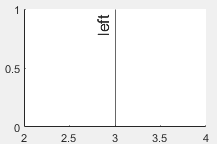'center'Center of the line. The label segments the line.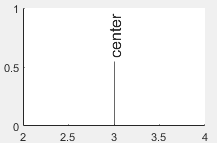Vertical alignment of the label with respect to the line, specified as one of the options in the table.

OptionDescriptionExample
'top'Top of the line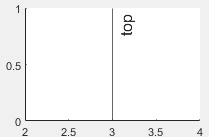'middle'Middle of the line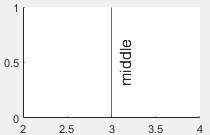'bottom'Bottom of the line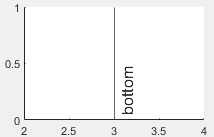Label orientation, specified as 'aligned' or 'horizontal'. Examples are shown in the table.

OrientationDescriptionExample
'aligned'Label has the same orientation as the line.'horizontal'Label is horizontal, regardless of the line orientation.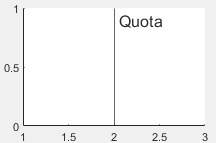## Algorithms

In a 3-D view of the axes, the constant line appears in the x-y plane at the midpoint of the z-axis limits. If the axes are rotated, then the constant line rotates accordingly.

### Properties

Introduced in R2018b